Processing ......FreeComputerBooks.com Links to Free Computer, Mathematics, Technical Books all over the World

Introduction to the Modeling and Analysis of Complex Systems
Top Free C Programming Books 🌠 - 100% Free or Open Source!
• Title: Introduction to the Modeling and Analysis of Complex Systems
• Author(s) Hiroki Sayama
• Publisher: Open SUNY Textbooks; Print edition; eBook (Creative Commons Licensed)
• Hardcover: 496 pages
• eBook: HTML and PDF
• Language: English
• ISBN-10: 1942341083
• ISBN-13: 978-1942341086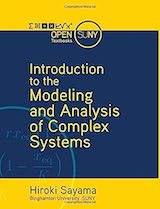Book Description

This textbook offers an accessible yet technically-oriented introduction to the modeling and analysis of complex systems. The topics covered include: fundamentals of modeling, basics of dynamical systems, discrete-time models, continuous-time models, bifurcations, chaos, cellular automata, continuous field models, static networks, dynamic networks, and agent-based models.

• N/A
Reviews, Ratings, and Recommendations: Related Book Categories: Read and Download Links:Similar Books:
•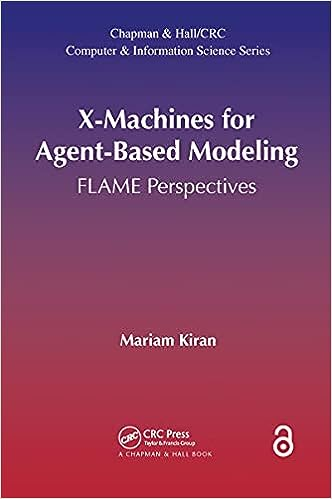X-Machines for Agent-Based Modeling (Mariam Kiran)

This book contains a comprehensive summary of the field, covers the basics of FLAME, and shows how concepts of X-Machines, can be stretched across multiple fields to produce Agent-Based Models.

•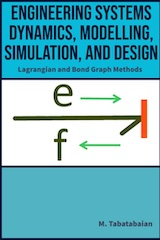Engineering Systems, Dynamics, Modelling, Simulation, and Design

This textbook is designed for engineering students and professionals in the field to support their understanding and application of these methods for modelling, simulation, and design of engineering systems.

•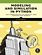Modeling and Simulation in Python (Allen B. Downey)

This book is an introduction to physical modeling using a computational approach with Python. You will learn how to use Python to accomplish many common scientific computing tasks: importing, exporting, and visualizing data; numerical analysis; etc.

•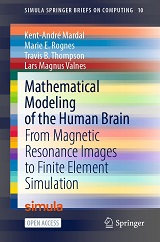Mathematical Modeling of the Human Brain

The book bridges common tools in medical imaging and neuroscience with the numerical solution of brain modelling PDEs, covers the basics of magnetic resonance imaging and quickly proceed to generating first FEniCS brain meshes from T1-weighted images.

•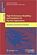Modelling and Simulation for Big Data Applications

Long considered important pillars of the scientific method, Modelling and Simulation have evolved from traditional discrete numerical methods to complex data-intensive continuous analytical optimisations.

•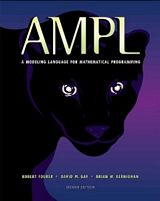AMPL: A Modeling Language for Mathematical Programming

This book is a complete guide to AMPL for modelers at all levels of experience. It begins with a tutorial on widely used linear programming models, and presents all of AMPL's features for linear programming with extensive examples.

•Modeling Reactive Systems With Statecharts (David Harel)

The book provides a detailed description of a set of languages for modelling reactive systems, which underlies the STATEMATE toolset. The approach is dominated by the language of Statecharts, used to describe behavior and activities.

•A Gentle Introduction to Numerical Simulations with Python

This book outlines the shortest possible path from no previous experience with programming to a set of skills that allows students to write simple programs for solving common mathematical problems with numerical methods in the context.

•Modeling Creativity - Case Studies in Python (Tom De Smedt)

This book is to model creativity using computational approaches in Python. The aim is to construct computer models that exhibit creativity in an artistic context, that is, that are capable of generating or evaluating an artwork (visual or linguistic), etc.

•Computer Simulation Techniques - The Definitive Introduction

This book addresses all the important aspects of a computer simulation study, including modeling, simulation languages, validation, input probability distribution, and analysis of simulation output data.

•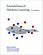Foundations of Machine Learning (Mehryar Mohri, et al)

This book is a general introduction to machine learning. It covers fundamental modern topics in machine learning while providing the theoretical basis and conceptual tools needed for the discussion and justification of algorithms.

•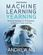Machine Learning Yearning (Andrew Ng)

You will learn how to align on ML strategies in a team setting, as well as how to set up development (dev) sets and test sets. After finishing this book, you will have a deep understanding of how to set technical direction for a machine learning project.

•Understanding Machine Learning: From Theory to Algorithms

Explains the principles behind the automated learning approach and the considerations underlying its usage. Provides an extensive theoretical account of the fundamental ideas underlying machine learning and the mathematical derivations.

Book Categories
 :All CategoriesTop Free BooksRecent BooksMiscellaneous BooksComputer EngineeringComputer LanguagesComputer ScienceData Science/DatabasesJava and Java EE (J2EE)Linux and UnixMathematicsMicrosoft and .NETMobile ComputingNetworking and CommunicationsSoftware EngineeringSpecial TopicsWeb Programming
Other Categories